Next: Integration Up: ALIAS-C++ Previous: Optimization

• La page de J-P. Merlet
• La page Présentation de HEPHAISTOS
• La page "Présentation" de l'INRIA

Subsections

# Continuation for one dimensional system

Although mostly devoted to zero-dimensional systems ALIAS still offers some possibilities to deal with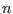-dimensional systems. The purpose of the algorithms here is to consider a system withparameter and to follow the branches of the solutions of the system when these parameters are varying between some bounds.

# Continuation 1D

## Mathematical background

Let consider a system ofequations in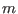unknowns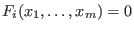and assume that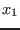may vary in the range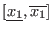and that it is considered as the parameter of this system. Using the solving algorithms of ALIAS (or any other method) we are able to determine the real roots of this system, if any, for a given value of the parameter, for example for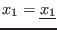. Let assume that we have found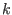real roots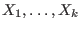. Assume now that we change the value ofto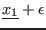. Using Kantorovitch theorem (see section 3.1.2) we are able to determine if Newton method with as initial estimate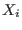will converge toward a solution of the new system and the radius of convergence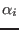such that the solution is unique in the ball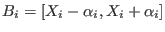(alternatively we may also use Moore theorem, see section 2.10). If this is the case and if the intersection of the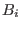is empty, then we are able to compute in a certified way the newsolutions of the new system. Otherwise we will repeat the procedure with a smaller value for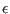until the method succeed. A failure case will be when the system become singular at a point (for example when 2 branches are collapsing). If this happen we will change the value ofuntil we find a new set of solutions which satisfy Kantorovitch theorem and start again the process.

This method enable to follow all the branches for which initial points have been found when solving the initial system. We may then store these points in an array, for example at regular step for the value of the parameter.

By default we use Kantorovitch theorem to follow the branches but by setting the global variable
ALIAS_Use_Kraw_Continuation to 1 we will use Moore test.

## Implementation

### Certified Newton

The procedure that allow to determine the next point on a branch is implemented as:


int Certified_Newton(int Nb_Var,int Dimension_Eq,int Nb_Branch,int Branch,
INTERVAL_VECTOR (* Func)(int,int,INTERVAL_VECTOR &),
INTERVAL_MATRIX (* Hessian)(int, int, INTERVAL_VECTOR &),
double Accuracy_Var,double Accuracy,
double *param,double delta_param,double min_delta_param,
int sens,MATRIX &Solution)

This procedure determine if it possible to find a new point on the branch numbered Branch among the Nb_Branch that have been found. In Solution we have all the solutions that have been found for the branches when the parameter value was param. The change in the parameter value will be at most sens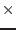delta_param and on exit of the procedure param will be set to the new value. sens indicates the direction of change in the parameter value: +1 for an increase, -1 for a decrease.

This procedure returns:

• -1, -4: even with a change of the parameter value of min_delta_param we cannot either distinguish two solutions or Kantorovitch has failed to give a positive answer. But this does not mean in general that a singularity occurs.
• -2: for systems having more equations than unknowns the solution that has been obtained with Newton for the square system of equations failed to cancel the remaining equations
• -3: Newton has not converged (should not occur)
• -10: singular point
• 1, 2: procedure has succeeded

### Procedure for following branches

This procedure takes as input a set ofsolutions of the system and will return points on thebranches. The branches will be followed until a given value for the parameter is reached or if Kantorovitch theorem is no more satisfied for some value of the parameter. It is implemented as:


int ALIAS_Start_Continuation(int m,int n,int NUM,
INTERVAL_MATRIX &Solutions,
INTERVAL_VECTOR (* IntervalFunction)(int,int,INTERVAL_VECTOR &),
INTERVAL_MATRIX (* IntervalHessian)(int,int,INTERVAL_VECTOR &),
double epsilon,double epsilonf,
double *z,double delta,double mindelta,
INTERVAL &Rangez,
int sens,
MATRIX &BRANCH,int *NBBRANCH)

the arguments being:
• m: number of unknowns
• n: number of equations
• NUM: the number of solutions of the system for the current value of the parameter z
• Solutions: the solutions of the system for the current value of the parameter z
• IntervalFunction: a function which return the interval vector evaluation of the equations, see note 2.3.4.3.
• IntervalGradient: a function which return the interval matrix of the jacobian of the equations, see note 2.4.2.2
• IntervalHessian: a function which return the interval matrix of the Hessian of the equations, see note 2.5.2.1
• epsilon: the maximal width of the box, see the note 2.3.4.6. If all the variable ranges have a width lower than this value and the interval evaluation of the equations contains all 0, then the set of ranges is considered to be a solution. But they will be not considered as a valid solution as they will not satisfy Kantorovitch theorem. Hence you must put here a very small value
• epsilonf: the maximal width of the equation intervals, see the note 2.3.4.6. This value will be used by the iterative Newton scheme to stop the iteration.
• z: the parameter of the system
• delta: if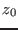is the first value of the parameter such that the system has solutions, then we will store in BRANCH the solutions for+delta,+2delta,...
• mindelta: if the algorithm is unable to find a Kantorovitch solution for the parameter value z+mindelta while a solution has been found for z, then the algorithm will assume that we are close to a singular point. Hence mindelta should have a small value
• Rangez: the range for the parameter z
• sens: either 1 or -1. If 1 the branch will be computed for increasing values of z, if -1 for decreasing values of z. This parameter should be chosen according to the largest number of solutions of the system for the lower and upper value of z
• BRANCH: the procedure will return an array of NBBRANCH lines and m+2 columns, which describe the points of the branch. In a line the first m+1 elements are the coordinates of the point and the m+2 elements is the number of the branch to which belong this point. The points are not ordered with respect to the branch number. The algorithm will take care of resizing BRANCH as needed, hence there is no need to give dimension for this parameter
• NBBRANCH: the total number of points in the array BRANCH.
The return code is:
• 1: the branches have been successfully determined
• -1, -2: the algorithm has failed to find a Kantorovitch solution for the current value of the parameter
• -3: failure in Newton method (should not occur)
• -10: for the current value of the parameter the jacobian is singular
• -20: sens is not 1 or -1
• -30: delta or mindelta is negative
Even for a negative return code the points in BRANCH are valid: the procedure will return the points that have computed on thebranches but is simply not able to determine the location of new branches that may have appeared.

### Full continuation procedure

This procedure will determine initial points on the branches of the system for the value of the parameter within some bounds and then follow the branches. It is implemented as

int ALIAS_Full_Continuation(int m,int n,
INTERVAL_VECTOR (* IntervalFunction)(int,int,INTERVAL_VECTOR &),
INTERVAL_MATRIX (* IntervalHessian)(int,int,INTERVAL_VECTOR &),
INTERVAL_VECTOR &Domain,
int M,
double epsilon,double epsilonf,
double *z,double delta,double mindelta,double mindz,
INTERVAL &Rangez,
int sens,
MATRIX &BRANCH,int *NBBRANCH)

The arguments are the same than for the previous procedure except for:
• Domain: the range for the m variable in which we will look for solutions of the system,
• M is the maximum number of boxes which may be stored (see the note 2.3.4.5)
• mindz: the accuracy with which the starting point for the branches will be determined: if the value of the parameter at which the branches will start is, then the system has no solution for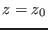-mindz. This is done by using a bisection on the parameter: if at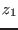the system has no solution and has a positive number of solution at+delta, then we will solve the system for+delta/2. At each time we will store the value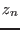of the parameter for which there is no solution of the system and the value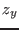for which we have solutions and we will stop the bisection on the parameter as soon as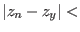mindz.
To determine the starting points of the branches this procedure uses the solving procedure with the Hessian (see section 2.5). As soon as initial valid solutions are found the ALIAS_Start_Continuation procedure is called until either a bound of the parameter range is reached, or a singularity occur. In the later case the solving procedure is called with an increased value for the parameter. This algorithm cannot find isolated points and may miss branches (see next section).

There is also another version of this program where you indicate just before Domain the solutions which have already been found. The syntax is


INTERVAL_MATRIX (* IntervalHessian)(int,int,INTERVAL_VECTOR &),
int NUM,
INTERVAL_MATRIX &Solutions,
INTERVAL_VECTOR &Domain


The return code for these procedures are:

•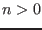: the number of branches found by the algorithm
• -1: no initial point have been found
• -10: Newton algorithm has failed (should not occur)
• -20: sens is not 1 or -1
• -30: delta or mindelta is negative

Finding the initial starting point with the accuracy mindz may be computer intensive. Hence the integer global variable ALIAS_Allow_Backtrack enable to disable this process if it is set to 0 (its default value is 1): in that case as soon as starting points have been found (hence at+delta) we will start following the branches.

In fact these procedures are special occurrences of another ALIAS procedure which has another argument right after the Hessian argument. Assume for example that you are considering a system which has one equation written as: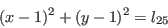where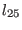is the parameter of the system and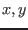the unknowns. When using the continuation method we have to define ranges for these unknowns in order to be able to solve the system of equations. Up to now we have indicated bounds that are constants but for the equation example it will be interesting to be able to specify that these bounds may change according to the value for the parameter using a simplification procedure. In our example clearly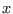and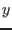cannot exceed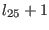and cannot be lower than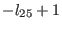(this is an application of the concept of 2B-consistency, see section 2.17). Hence we may specify right after the Hessian argument the name of a procedure, for example Range, that is able to update the bounds on the unknowns according to the value of the parameter (or any other variable that may play a role). The syntax of the procedure Range is:
INTERVAL_VECTOR Range(double z, INTERVAL_VECTOR &Variable)

where z is parameter value and Variable the current set of ranges for the unknowns. This procedure must return a set of ranges for the unknowns (be careful to check that the returned ranges are included in the initial ranges).

Note also that the ALIAS-Maple package offers a procedure that uses the method described in section 2.14 for finding the initial starting points of the branches: this method is efficient if the equations include linear terms in the unknowns.

### Missed branches

Clearly there is a major problem with the method we are proposing: we may miss some branches. For example imagine that a system hasroots for the initial value of the parameter, but will have more thansolutions for another value of the parameter, meaning that new branches appear: our algorithm will find only theinitial branches. There are two methods that can be used to find the correct number of branches. The first one is simply to start following the branches with as initial value for the parameter the one among the highest or smallest value having led to the maximum number of solution. There is also another mechanism that enable to avoid missing branches. Assume that the solving procedure has determined for some initial value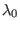of the parametersolutions to the system and that at some point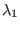the continuation method has failed: the solving procedure is called and determine that the system has now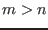solutions. This mean that for some parameter value betweenandwe have missed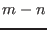branches. At such point, called problem point it would be interesting to backtrack i.e. to start again a continuation process with as initial point for the branches thesolutions obtained forand a value for Sens which is the opposite of the initial value. This is what is done by the procedure which may store up to 10 problem points. As this process may be computer intensive it is possible to disable it by setting the integer global variable ALIAS_Problem_Continuation to -1 (it's default value is 0, which mean that the process is enabled).

## Example

Let consider the system: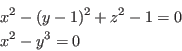The purpose of this example is to write the points of the branches of this system in files whose name is BRANCH followed by the branch number. The main file is written as:

#include "Functions.h"
#include "Vector.h"
#include "IntervalVector.h"
#include "IntervalMatrix.h"
#include "IntegerMatrix.h"
double z;
//F,J,H are the equation, jacobian and hessian procedures
#include "F.C"
#include "J.C"
#include "H.C"

int main()
{
MATRIX BRANCH;
INTERVAL RANGE;
int NUM,i,j,NB_BRANCH;
char texte;
double delta=0.05;
double min_delta=1.e-6;
double mindz=1.e-4;
ostream out;

int Nb_Var=2;
int Nb_Eq=2;
Domain(1)=INTERVAL(-100,100);
Domain(2)=INTERVAL(-100,100);
RANGE=INTERVAL(0.2);
NUM=ALIAS_Full_Continuation(Nb_Var,Nb_Eq,F,J,H,Domain,1.e-6,1.e-6,&z,
delta,min_delta,mindz,RANGE,1,BRANCH,&NB_BRANCH);

//order the branch and write the result in the file
for(i =1;i<=NUM;i++)
{
sprintf(texte,"BRANCH%d",i);
out.open(texte,ios::out);
for(j =1;j<=NB_BRANCH;j++)
{
if(BRANCH(j,Nb_Var+2)!=i)continue;
out<<BRANCH(j,1)<<" "<<BRANCH(j,2)<<" "<<BRANCH(j,3)<<endl;
}
out.close();
}
}Next: Integration Up: ALIAS-C++ Previous: Optimization